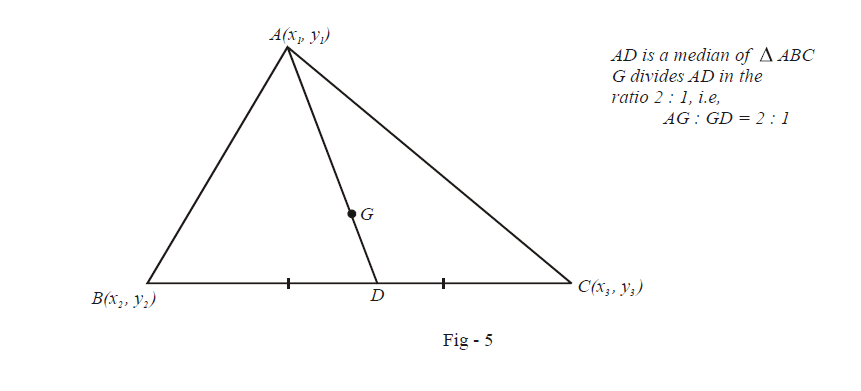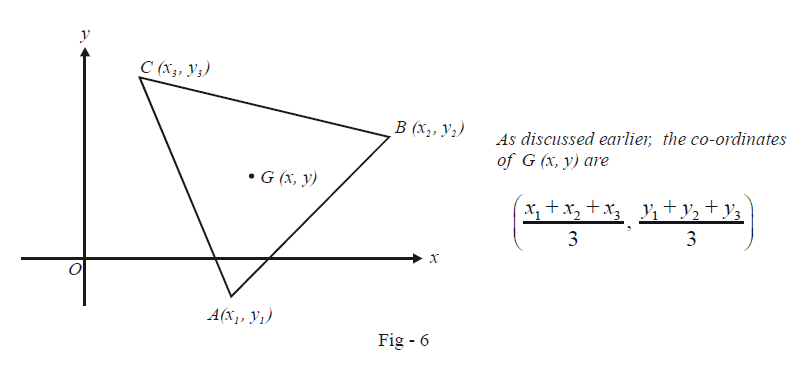# Examples on Distance and Section Formulae

Go back to  'Straight Lines'

Example – 1

Find the co-ordinates of the centroid of a triangle with the vertices $$A\left( {{x}_{1}},{{y}_{1}} \right),\,\,B\left( {{x}_{2}},{{y}_{2}} \right)\,\,\text{and}\,\,C\left( {{x}_{3}},{{y}_{3}} \right).$$

Solution: To determine the centroid, we will borrow a result from plane geometry that you might remember from high school: the centroid divides any median in the ratio 2 : 1.The co-ordinates of D, the mid-point of BC, are  \begin{align}\left( \frac{{{x}_{2}}+{{x}_{3}}}{2},\frac{{{y}_{2}}+{{y}_{3}}}{2} \right)\end{align} . Since  $$AG:GD=2:1,$$ we have the co-ordinates of G by the section formula as

\begin{align} & \;G\equiv \frac{2\left(\begin{align} \frac{{{x}_{2}}+{{x}_{3}}}{2} \end{align}\right)+1.{{x}_{1}}}{2+1},\,\,\,\frac{2\left(\begin{align} \frac{{{y}_{2}}+{{y}_{3}}}{2}\end{align} \right)+1.{{y}_{1}}}{2+1} \\ & \quad\equiv \frac{{{x}_{1}}+{{x}_{2}}+{{x}_{3}}}{3},\,\,\,\frac{{{y}_{1}}+{{y}_{2}}+{{y}_{3}}}{3} \end{align}

The expression for the centroid confirms the obvious fact that the co-ordinates of the centroid are ‘symmetric’ with respect to the co-ordinates of the three vertices of the triangle.

Example – 2

G is the centroid of triangle ABC. If O is any other point in the plane, prove that

$O{{A}^{2}}+O{{B}^{2}}+O{{C}^{2}}=G{{A}^{2}}+G{{B}^{2}}+G{{C}^{2}}+3\,G{{O}^{2}}.$

Solution: There’s no loss of generality in taking O as the origin of our reference axis since even if we are given O to be a non-origin point, we can always translate the axes (keeping the triangle ABC unchanged) so that its origin coincides with O. Note that this operation will have no effect on the lengths $$OA,\,\,OB,\,\,OC,\,\,OG,\,\,GA,\,\,GB,\,\,GC$$ etc. However, the expressions for distances will become much more simplified (In co-ordinate geometry, you will be required to follow such steps often, so that the expressions you are to deal with can be kept as simple as possible).

Now, we assume some co-ordinates for A, B and C as shown in the figure below:We have,

$O{{A}^{2}}+\,O{{B}^{2}}+\,\,O{{C}^{2}}\,\,\,\,=\,\,\,\,\,x_{1}^{2}+y_{1}^{2}+x_{2}^{2}+y_{2}^{2}+x_{3}^{2}+y_{3}^{2}\qquad \qquad ...\text{ }\left( 1 \right)$

while
\begin{align} & G{{A}^{2}}+G{{B}^{2}}+G{{C}^{2}}+3G{{O}^{2}}\,\,\,\,=\,\,\,\,\,{{\left( x-{{x}_{1}} \right)}^{2}}+{{\left( y-{{y}_{1}} \right)}^{2}}+{{\left( x-{{x}_{2}} \right)}^{2}}+{{\left( y-{{y}_{2}} \right)}^{2}}\\&\qquad\qquad\qquad\qquad\qquad\qquad\qquad+\,{{\left( x-{{x}_{3}} \right)}^{2}}+{{\left( y-{{y}_{3}} \right)}^{2}}+3\left( {{x}^{2}}+{{y}^{2}} \right) \\&\qquad\qquad\qquad\qquad\qquad\qquad\quad=\,\,\,x_{1}^{2}+x_{2}^{2}+x_{3}^{2}+y_{1}^{2}+y_{2}^{2}+y_{3}^{2}\qquad \qquad ...\text{ }\left( 2 \right) \\ \end{align}

Comparing (1) and (2), we see that the two expressions are indeed equal